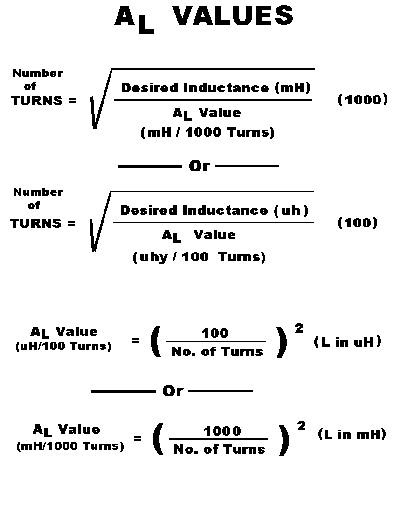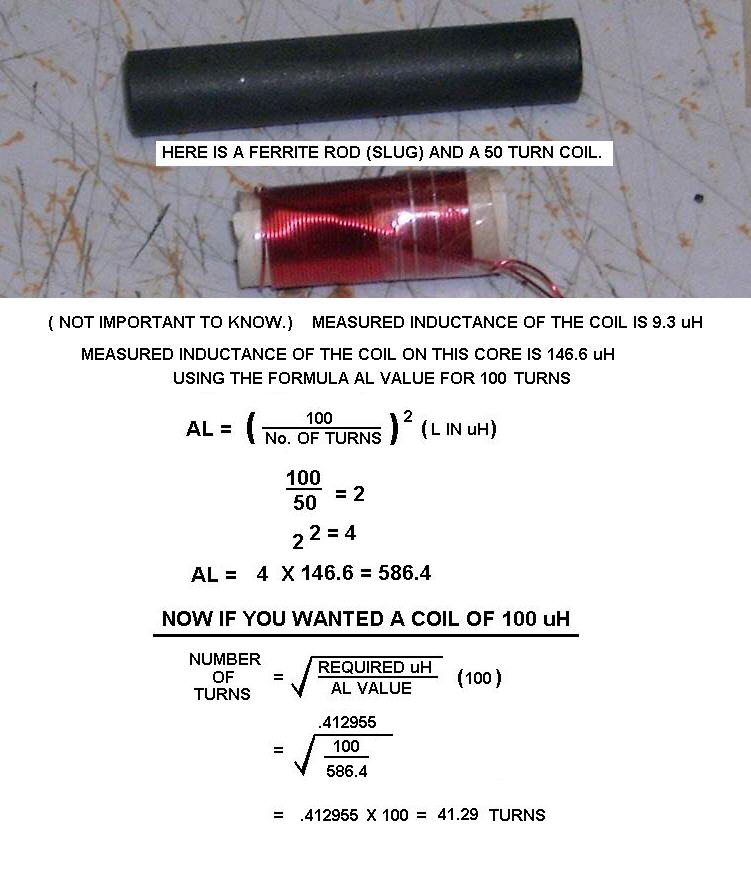# Ferrite Inductors and AL Values.

## Or for that matter, Iron Core Inductors Also.

Created: "May 3 2005"
Revised: "Nov, 30 2009"

```Knowing the AL Values of a Ferrite, (or Other Core Materials) Allows a person to Easily
calculate the turns required for winding different Values of Inductance on Specific Inductor Cores.

However in order to Determine the AL Value of a Ferrite core,
You "Do Need an Inductance Meter", Or some other method of Determining the Inductance.
A Signal Generator and a Oscilloscope would work, using a Known Capacitor to create a Resonance.

To Start:
Wind a Specific Number of turns on the Unknown Core.
Note: More Turns Results in a More accurate answer.

I usually Recommend 100 Turns, but if accuracy isn't important, 10 or 20 turns will do.
Than MEASURE the Inductance.

Now using One of the Two "AL Value Formulas" in the Picture Below,
You can determine the AL Value in uH or mH.

As an Example, I wound 20 turns of wire on a Ferrite Rod.
The Measured Inductance was 22.4 uH.

100 / 20.0 = 5.0
5.02 = 25.0
25.0 X 22.4 = An AL Value of 560

Now lets assume you want a 100 uh Choke, based on the Above AL Value.
Using the Appropriate Formula for the Number of Turns:

100 / 560 = 0.1785

The Square Root of 0.1785 = 0.4226

0.4226 X 100 = 42 Turns

However, The Actual Number of turns for 100 uH is 45 Turns
Or at least that was what I had to wind on this particular Ferrite Rod, in order to Measure 100 uH.

If I had of Initally Used "100 Turns", I would have acquired a More Accurate " AL Value ".
And I'm sure it would have come out closer.

I use these formulas Quite a lot when I acquire Batches of Unknown Ferrites.
I usually Test Two in a batch by just slipping the coil off of one and onto the next.
In Most cases the results are pretty consistant.

I than Mark the batches and when I need to use one, I already have the Necessary
AL Value to make whatever I want.

IMPORTANT:
If you are using a Pot Core, E-Core or U Core that is Gapped or one you want gapped.,
You "Must" Gap it for your Inital Winding, Or you will NOT get a Correct AL Value."Formulas""With a Coil Picture"

"Back to My Projects Page"

```

```All Imformation in this Article is "Copyright protected".